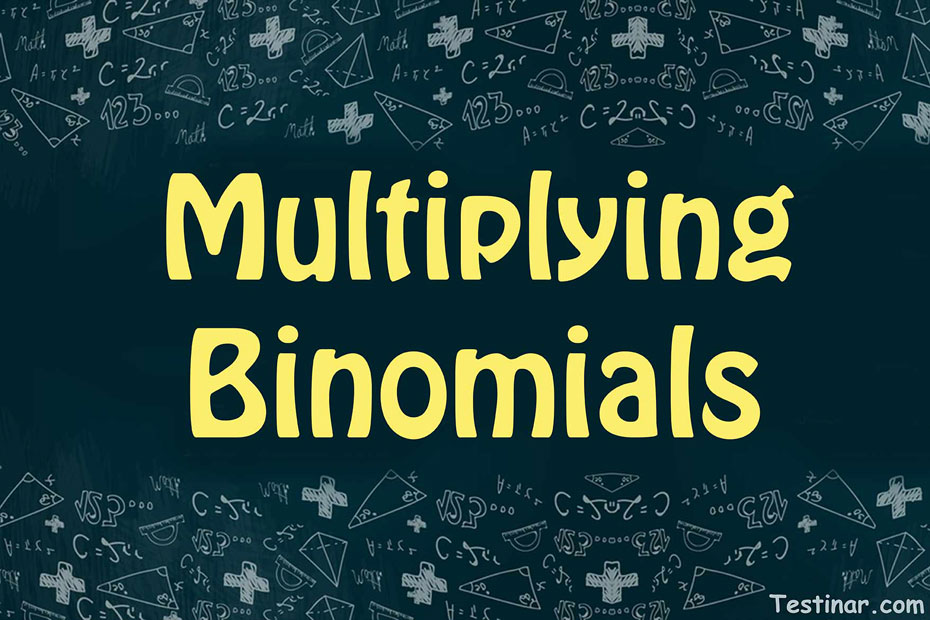## How to Multiply Binomials

The phrase "binomial," as the name implies, is made up of the terms "bi" and "nomial," which relate to a variety of expressions. A binomial is an algebraic or mathematical phrase consisting of two terms linked by a mathematical operation. Furthermore, the binomial terms may include constants, variables, or a mixed term with a co-efficient. Furthermore, the variable's power (let's say $$x$$) in a binomial should be an integer, not a fractional power like the squared or cube root.
For example, $$2x^{\frac{1}{3}} \ + \ 3x$$ is not a binomial as the first term has a fraction power of $$x$$.

### Degree of a Binomial

Unlike a polynomial, the degree of a binomial is pretty simple to find. Since a binomial consists of only two terms, hence its degree would obviously be the greatest exponent power of that variable. So, for example in the term $$4x^3 \ + \ 3x^2$$, we can see that the greatest degree of $$x$$ is $$3$$. So, the degree of the binomial is considered as $$3$$.
Now, for a binomial expression with multiple variables, the case becomes quite complex. So, for example, let’s take the multivariable binomial expression $$3x^2y^3 \ + \ 4x^3$$. Now, here we can clearly see that the binomial has 2 different variables as $$x$$ and $$y$$. So, what we do here is add up the exponent powers of the separate variables. So, in this case the addition comes as $$2 \ + \ 3 \ = \ 5$$. Hence, the degree of this monomial is $$5$$ (we do not consider the degree of $$4x^3$$ i.e., $$3$$ since it is lower than the multivariable term degree).

### Multiplying Binomials

While multiplying binomials, take note of the following steps:

• When you are multiplying binomials, always remember the exponents rule. Multiplication always leads to addition of exponent terms of the same variables.
• Next, you should always use the distributive property while multiplying binomial.
• Next, apply the FOIL rule which is First Out Last In.

Let’s understand the multiplication of binomials by the following example:

• $$(−9x \ − \ 1)(−4x \ + \ 4) \ = \ 36x^2 \ + \ (−36)x \ + \ 4x \ + \ (−4) \ = \ 36x^2 \ − \ 32x \ – \ 4$$

### Exercises for Multiplying Binomials

1) $$(-9x \ - \ 1)(-4x \ + \ 4) =$$

2) $$(-9x \ - \ 8)(3x \ + \ 3) =$$

3) $$(-6x \ - \ 9)(2x \ + \ 5) =$$

4) $$(-5x \ - \ 4)(1x \ + \ 1) =$$

5) $$(-3x \ - \ 2)(-6x \ + \ 5) =$$

6) $$(-4x \ - \ 7)(5x \ + \ 4) =$$

7) $$(-2x \ - \ 5)(-4x \ + \ 4) =$$

8) $$(-1x \ - \ 10)(-6x \ + \ 2) =$$

9) $$(-10x \ - \ 3)(-5x \ + \ 1) =$$

10) $$(-7x \ - \ 6)(-2x \ + \ 2) =$$

1) $$(-9x \ - \ 1)(-4x \ + \ 4) =$$$$\ \color{red}{36x^2 \ + \ (-36)x \ + \ 4x \ + \ (-4)}$$$$\ \color{red}{= \ 36x^2 \ - \ 32x \ - \ 4}$$
2) $$(-9x \ - \ 8)(3x \ + \ 3) =$$$$\ \color{red}{-27x^2 \ + \ (-27)x \ + \ (-24)x \ + \ (-24)}$$$$\ \color{red}{= \ -27x^2 \ - \ 51x \ - \ 24}$$
3) $$(-6x \ - \ 9)(2x \ + \ 5) =$$$$\ \color{red}{-12x^2 \ + \ (-30)x \ + \ (-18)x \ + \ (-45)}$$$$\ \color{red}{= \ -12x^2 \ - \ 48x \ - \ 45}$$
4) $$(-5x \ - \ 4)(1x \ + \ 1) =$$$$\ \color{red}{-5x^2 \ + \ (-5)x \ + \ (-4)x \ + \ (-4)}$$$$\ \color{red}{= \ -5x^2 \ - \ 9x \ - \ 4}$$
5) $$(-3x \ - \ 2)(-6x \ + \ 5) =$$$$\ \color{red}{18x^2 \ + \ (-15)x \ + \ 12x \ + \ (-10)}$$$$\ \color{red}{= \ 18x^2 \ - \ 3x \ - \ 10}$$
6) $$(-4x \ - \ 7)(5x \ + \ 4) =$$$$\ \color{red}{-20x^2 \ + \ (-16)x \ + \ (-35)x \ + \ (-28)}$$$$\ \color{red}{= \ -20x^2 \ - \ 51x \ - \ 28}$$
7) $$(-2x \ - \ 5)(-4x \ + \ 4) =$$$$\ \color{red}{8x^2 \ + \ (-8)x \ + \ 20x \ + \ (-20)}$$$$\ \color{red}{= \ 8x^2 \ + \ 12x \ - \ 20}$$
8) $$(-1x \ - \ 10)(-6x \ + \ 2) =$$$$\ \color{red}{6x^2 \ + \ (-2)x \ + \ 60x \ + \ (-20)}$$$$\ \color{red}{= \ 6x^2 \ + \ 58x \ - \ 20}$$
9) $$(-10x \ - \ 3)(-5x \ + \ 1) =$$$$\ \color{red}{50x^2 \ + \ (-10)x \ + \ 15x \ + \ (-3)}$$$$\ \color{red}{= \ 50x^2 \ + \ 5x \ - \ 3}$$
10) $$(-7x \ - \ 6)(-2x \ + \ 2) =$$$$\ \color{red}{14x^2 \ + \ (-14)x \ + \ 12x \ + \ (-12)}$$$$\ \color{red}{= \ 14x^2 \ - \ 2x \ - \ 12}$$

## Multiplying Binomials Practice Quiz

### DAT Quantitative Reasoning Preparation 2020 – 2021

$18.99$13.99

### TABE 11 & 12 Math in 10 Days

$24.99$13.99

### SSAT Middle Level Math Comprehensive Prep Bundle

$89.99$49.99

### SSAT Middle Level Mathematics Workbook

$16.99$11.99